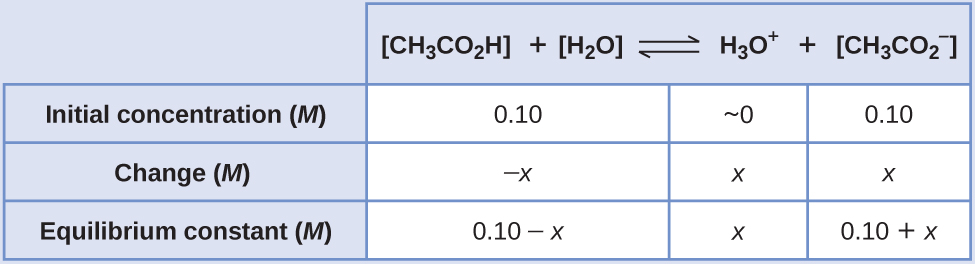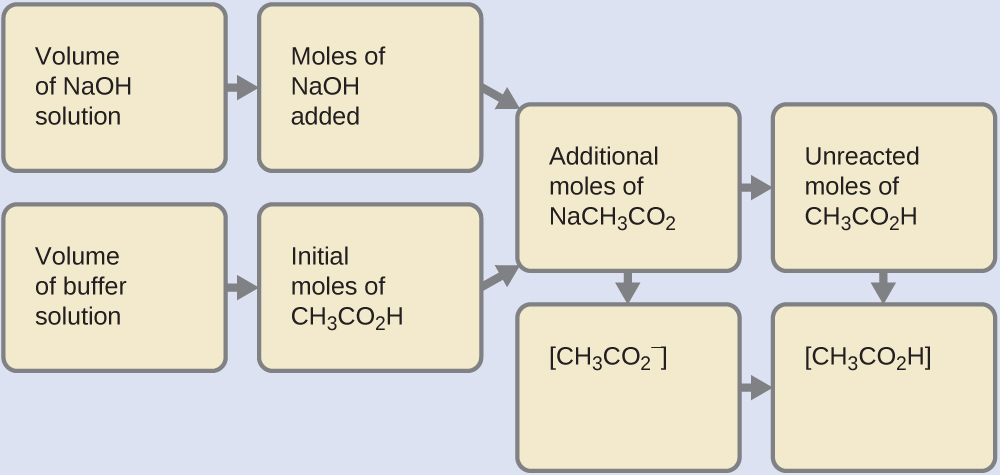# 8.6 Buffers  (Page 2/9)

 Page 2 / 9

## Ph changes in buffered and unbuffered solutions

Acetate buffers are used in biochemical studies of enzymes and other chemical components of cells to prevent pH changes that might change the biochemical activity of these compounds.

(a) Calculate the pH of an acetate buffer that is a mixture with 0.10 M acetic acid and 0.10 M sodium acetate.

## Solution

To determine the pH of the buffer solution we use a typical equilibrium calculation (as illustrated in earlier Examples):1. Determine the direction of change. The equilibrium in a mixture of H 3 O + , ${\text{CH}}_{3}{\text{CO}}_{2}{}^{\text{−}},$ and CH 3 CO 2 H is:
${\text{CH}}_{3}{\text{CO}}_{2}\text{H}\left(aq\right)+{\text{H}}_{2}\text{O}\left(l\right)\phantom{\rule{0.2em}{0ex}}⇌\phantom{\rule{0.2em}{0ex}}{\text{H}}_{3}{\text{O}}^{\text{+}}\left(aq\right)+{\text{CH}}_{3}{\text{CO}}_{2}{}^{\text{−}}\left(aq\right)$

The equilibrium constant for CH 3 CO 2 H is not given, so we look it up in Appendix H : K a = 1.8 $×$ 10 −5 . With [CH 3 CO 2 H] = $\left[{\text{CH}}_{3}{\text{CO}}_{2}{}^{\text{−}}\right]$ = 0.10 M and [H 3 O + ] = ~0 M , the reaction shifts to the right to form H 3 O + .
2. Determine x and equilibrium concentrations . A table of changes and concentrations follows:3. Solve for x and the equilibrium concentrations. We find:
$x=1.8\phantom{\rule{0.2em}{0ex}}×\phantom{\rule{0.2em}{0ex}}{10}^{-5}M$

and
$\left[{\text{H}}_{3}{\text{O}}^{\text{+}}\right]\phantom{\rule{0.2em}{0ex}}=0+x=1.8\phantom{\rule{0.2em}{0ex}}×\phantom{\rule{0.2em}{0ex}}{10}^{-5}\phantom{\rule{0.2em}{0ex}}M$

Thus:
$\text{pH}=\text{−log}\left[{\text{H}}_{3}{\text{O}}^{\text{+}}\right]\phantom{\rule{0.2em}{0ex}}=\phantom{\rule{0.2em}{0ex}}\text{−log}\left(1.8\phantom{\rule{0.2em}{0ex}}×\phantom{\rule{0.2em}{0ex}}{10}^{-5}\right)$

$=4.74$

4. Check the work . If we calculate all calculated equilibrium concentrations, we find that the equilibrium value of the reaction coefficient, Q = K a .

(b) Calculate the pH after 1.0 mL of 0.10 M NaOH is added to 100 mL of this buffer, giving a solution with a volume of 101 mL.

First, we calculate the concentrations of an intermediate mixture resulting from the complete reaction between the acid in the buffer and the added base. Then we determine the concentrations of the mixture at the new equilibrium:1. Determine the moles of NaOH. One milliliter (0.0010 L) of 0.10 M NaOH contains:
$0.0010\phantom{\rule{0.2em}{0ex}}\overline{)\text{L}}\phantom{\rule{0.2em}{0ex}}×\phantom{\rule{0.2em}{0ex}}\left(\phantom{\rule{0.2em}{0ex}}\frac{0.10\phantom{\rule{0.2em}{0ex}}\text{mol NaOH}}{1\phantom{\rule{0.2em}{0ex}}\overline{)\text{L}}}\right)\phantom{\rule{0.2em}{0ex}}\phantom{\rule{0.2em}{0ex}}=1.0\phantom{\rule{0.2em}{0ex}}×\phantom{\rule{0.2em}{0ex}}{10}^{-4}\phantom{\rule{0.2em}{0ex}}\text{mol NaOH}$
2. Determine the moles of CH 2 CO 2 H. Before reaction, 0.100 L of the buffer solution contains:
$0.100\phantom{\rule{0.2em}{0ex}}\overline{)\text{L}}\phantom{\rule{0.2em}{0ex}}×\phantom{\rule{0.2em}{0ex}}\left(\phantom{\rule{0.2em}{0ex}}\frac{0.100\phantom{\rule{0.2em}{0ex}}\text{mol}\phantom{\rule{0.2em}{0ex}}{\text{CH}}_{3}{\text{CO}}_{2}\text{H}}{1\phantom{\rule{0.2em}{0ex}}\overline{)\text{L}}}\right)\phantom{\rule{0.2em}{0ex}}\phantom{\rule{0.2em}{0ex}}=1.00\phantom{\rule{0.2em}{0ex}}×\phantom{\rule{0.2em}{0ex}}{10}^{-2}\phantom{\rule{0.2em}{0ex}}\text{mol}\phantom{\rule{0.2em}{0ex}}{\text{CH}}_{3}{\text{CO}}_{2}\text{H}$
3. Solve for the amount of NaCH 3 CO 2 produced. The 1.0 $×$ 10 −4 mol of NaOH neutralizes 1.0 $×$ 10 −4 mol of CH 3 CO 2 H, leaving:
$\left(1.0\phantom{\rule{0.2em}{0ex}}×\phantom{\rule{0.2em}{0ex}}{10}^{-2}\right)\phantom{\rule{0.2em}{0ex}}-\phantom{\rule{0.2em}{0ex}}\left(0.01\phantom{\rule{0.2em}{0ex}}×\phantom{\rule{0.2em}{0ex}}{10}^{-2}\right)\phantom{\rule{0.2em}{0ex}}=0.99\phantom{\rule{0.2em}{0ex}}×\phantom{\rule{0.2em}{0ex}}{10}^{-2}\phantom{\rule{0.2em}{0ex}}\text{mol}\phantom{\rule{0.2em}{0ex}}{\text{CH}}_{3}{\text{CO}}_{2}\text{H}$

and producing 1.0 $×$ 10 −4 mol of NaCH 3 CO 2 . This makes a total of:
$\left(1.0\phantom{\rule{0.2em}{0ex}}×\phantom{\rule{0.2em}{0ex}}{10}^{-2}\right)+\left(0.01\phantom{\rule{0.2em}{0ex}}×\phantom{\rule{0.2em}{0ex}}{10}^{-2}\right)\phantom{\rule{0.2em}{0ex}}=1.01\phantom{\rule{0.2em}{0ex}}×\phantom{\rule{0.2em}{0ex}}{10}^{-2}\text{mol}\phantom{\rule{0.2em}{0ex}}{\text{NaCH}}_{3}{\text{CO}}_{2}$
4. Find the molarity of the products. After reaction, CH 3 CO 2 H and NaCH 3 CO 2 are contained in 101 mL of the intermediate solution, so:
$\left[{\text{CH}}_{3}{\text{CO}}_{2}\text{H}\right]\phantom{\rule{0.2em}{0ex}}=\phantom{\rule{0.2em}{0ex}}\frac{9.9\phantom{\rule{0.2em}{0ex}}×\phantom{\rule{0.2em}{0ex}}{10}^{-3}\phantom{\rule{0.2em}{0ex}}\text{mol}}{0.101\phantom{\rule{0.2em}{0ex}}\text{L}}\phantom{\rule{0.2em}{0ex}}=0.098\phantom{\rule{0.2em}{0ex}}M$

$\left[{\text{NaCH}}_{3}{\text{CO}}_{2}\right]\phantom{\rule{0.2em}{0ex}}=\phantom{\rule{0.2em}{0ex}}\frac{1.01\phantom{\rule{0.2em}{0ex}}×\phantom{\rule{0.2em}{0ex}}{10}^{-2}\phantom{\rule{0.2em}{0ex}}\text{mol}}{0.101\phantom{\rule{0.2em}{0ex}}\text{L}}\phantom{\rule{0.2em}{0ex}}=0.100\phantom{\rule{0.2em}{0ex}}M$

Now we calculate the pH after the intermediate solution, which is 0.098 M in CH 3 CO 2 H and 0.100 M in NaCH 3 CO 2 , comes to equilibrium. The calculation is very similar to that in part (a) of this example:This series of calculations gives a pH = 4.75. Thus the addition of the base barely changes the pH of the solution ( [link] ).

(c) For comparison, calculate the pH after 1.0 mL of 0.10 M NaOH is added to 100 mL of a solution of an unbuffered solution with a pH of 4.74 (a 1.8 $×$ 10 −5 - M solution of HCl). The volume of the final solution is 101 mL.

## Solution

This 1.8 $×$ 10 −5 - M solution of HCl has the same hydronium ion concentration as the 0.10- M solution of acetic acid-sodium acetate buffer described in part (a) of this example. The solution contains:

$0.100\phantom{\rule{0.2em}{0ex}}\text{L}\phantom{\rule{0.2em}{0ex}}×\phantom{\rule{0.2em}{0ex}}\left(\phantom{\rule{0.2em}{0ex}}\frac{1.8\phantom{\rule{0.2em}{0ex}}×\phantom{\rule{0.2em}{0ex}}{10}^{-5}\text{mol HCl}}{1\phantom{\rule{0.2em}{0ex}}\text{L}}\right)\phantom{\rule{0.2em}{0ex}}\phantom{\rule{0.2em}{0ex}}=1.8\phantom{\rule{0.2em}{0ex}}×\phantom{\rule{0.2em}{0ex}}{10}^{-6}\text{mol HCl}$

As shown in part (b), 1 mL of 0.10 M NaOH contains 1.0 $×$ 10 −4 mol of NaOH. When the NaOH and HCl solutions are mixed, the HCl is the limiting reagent in the reaction. All of the HCl reacts, and the amount of NaOH that remains is:

where we get a research paper on Nano chemistry....?
nanopartical of organic/inorganic / physical chemistry , pdf / thesis / review
Ali
what are the products of Nano chemistry?
There are lots of products of nano chemistry... Like nano coatings.....carbon fiber.. And lots of others..
learn
Even nanotechnology is pretty much all about chemistry... Its the chemistry on quantum or atomic level
learn
da
no nanotechnology is also a part of physics and maths it requires angle formulas and some pressure regarding concepts
Bhagvanji
hey
Giriraj
Preparation and Applications of Nanomaterial for Drug Delivery
revolt
da
Application of nanotechnology in medicine
what is variations in raman spectra for nanomaterials
ya I also want to know the raman spectra
Bhagvanji
I only see partial conversation and what's the question here!
what about nanotechnology for water purification
please someone correct me if I'm wrong but I think one can use nanoparticles, specially silver nanoparticles for water treatment.
Damian
yes that's correct
Professor
I think
Professor
Nasa has use it in the 60's, copper as water purification in the moon travel.
Alexandre
nanocopper obvius
Alexandre
what is the stm
is there industrial application of fullrenes. What is the method to prepare fullrene on large scale.?
Rafiq
industrial application...? mmm I think on the medical side as drug carrier, but you should go deeper on your research, I may be wrong
Damian
How we are making nano material?
what is a peer
What is meant by 'nano scale'?
What is STMs full form?
LITNING
scanning tunneling microscope
Sahil
how nano science is used for hydrophobicity
Santosh
Do u think that Graphene and Fullrene fiber can be used to make Air Plane body structure the lightest and strongest. Rafiq
Rafiq
what is differents between GO and RGO?
Mahi
what is simplest way to understand the applications of nano robots used to detect the cancer affected cell of human body.? How this robot is carried to required site of body cell.? what will be the carrier material and how can be detected that correct delivery of drug is done Rafiq
Rafiq
if virus is killing to make ARTIFICIAL DNA OF GRAPHENE FOR KILLED THE VIRUS .THIS IS OUR ASSUMPTION
Anam
analytical skills graphene is prepared to kill any type viruses .
Anam
Any one who tell me about Preparation and application of Nanomaterial for drug Delivery
Hafiz
what is Nano technology ?
write examples of Nano molecule?
Bob
The nanotechnology is as new science, to scale nanometric
brayan
nanotechnology is the study, desing, synthesis, manipulation and application of materials and functional systems through control of matter at nanoscale
Damian
Is there any normative that regulates the use of silver nanoparticles?
what king of growth are you checking .?
Renato
What fields keep nano created devices from performing or assimulating ? Magnetic fields ? Are do they assimilate ?
why we need to study biomolecules, molecular biology in nanotechnology?
?
Kyle
yes I'm doing my masters in nanotechnology, we are being studying all these domains as well..
why?
what school?
Kyle
biomolecules are e building blocks of every organics and inorganic materials.
Joe
how do you find theWhat are the wavelengths and energies per photon of two lines
The eyes of some reptiles are sensitive to 850 nm light. If the minimum energy to trigger the receptor at this wavelength is 3.15 x 10-14 J, what is the minimum number of 850 nm photons that must hit the receptor in order for it to be triggered?
A teaspoon of the carbohydrate sucrose contains 16 calories, what is the mass of one teaspoo of sucrose if the average number of calories for carbohydrate is 4.1 calories/g?
4. On the basis of dipole moments and/or hydrogen bonding, explain in a qualitative way the differences in the boiling points of acetone (56.2 °C) and 1-propanol (97.4 °C), which have similar molar masses
Calculate the bond order for an ion with this configuration: (?2s)2(??2s)2(?2px)2(?2py,?2pz)4(??2py,??2pz)3
Which of the following will increase the percent of HF that is converted to the fluoride ion in water? (a) addition of NaOH (b) addition of HCl (c) addition of NaFBy Anh DaoBy OpenStaxBy Vongkol HENGBy IES PortalBy Brooke DelaneyBy Jonathan LongBy OpenStaxBy Rohini AjayBy OpenStaxBy OpenStax